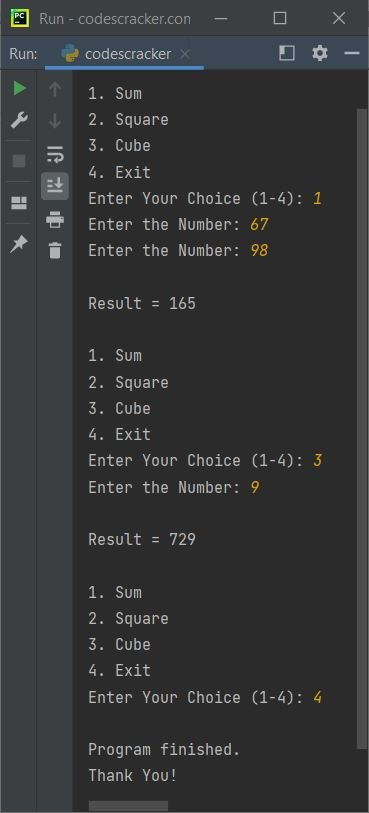# Module in Python (with Examples)

In Python, a module is essentially a file that contains a set of functions and/or objects. For example:

```def greet():
print("Hey,")
print("a warm welcome from my side.")```

Save this code in a file with the extension ".py," such as mod.py. Put this file in the current directory, the directory where the Python program (source code) is available. Here is the snapshot of the current directory, in my case:Now create a program that imports the newly created module mod.py to use functions defined inside that module in the program. For example:

```import mod

mod.greet()```

This will produce the following output:

```Hey,
a warm welcome from my side.```

Note: The import statement or keyword is used to load a module in Python.

Important: The module file must be saved with the ".py" extension.

## Why do we need to create a module in Python?

If you're creating an application in Python that has an extensive number of functions, variables, and/or other objects, then it's better to put some or all of those functions, variables, and/or other objects inside a separate file called a module.

It becomes easy to redefine or edit the module file, which contains some part of the actual program, without opening the source code file. Also, the source code file will get smaller and become easier to handle.

Note: Modules some time helps to organize the code. Furthermore, after grouping the related codes into module(s), the long program becomes shorter.

## How to Use a Module in Python

To use a module in Python, first load the module using the "import" keyword. Here is the syntax:

`import module_name`

If you want to load only a particular function of a module, then use "import" along with the from keyword in this way:

`from module_name import function_name`

After loading the module in your program, use the functions defined in that module in this way:

`module_name.function_name`

Note: When using a function defined in a module, it is required to bind the function to the module. Since in some large applications there may be multiple functions with the same name defined in different modules, And if those modules are loaded inside the same program, then directly calling the function will raise an error.

## Python Module Example: Create and Use a Module in a Program

As previously stated, a module is a file with the extension ".py" that contains some code blocks. For example, save the following block of codes in a file called "mymodule.py":

```def sum(a, b):
return a+b

def sqr(x):
return x*x

def cub(x):
return x*x*x

def msg():
print("Enter the Number: ", end="")```

And to use this module, create another program. For example:

```import mymodule

choice = 1
while 1 <= choice <= 4:
print("\n1. Sum")
print("2. Square")
print("3. Cube")
print("4. Exit")
print("Enter Your Choice (1-4): ", end="")
choice = int(input())

if choice == 1:
mymodule.msg()
numOne = int(input())
mymodule.msg()
numTwo = int(input())
res = mymodule.sum(numOne, numTwo)
print("\nResult =", res)

elif choice == 2:
mymodule.msg()
num = int(input())
res = mymodule.sqr(num)
print("\nResult =", res)

elif choice == 3:
mymodule.msg()
num = int(input())
res = mymodule.cub(num)
print("\nResult =", res)

elif choice == 4:
choice = 5

else:
choice = 1
print("\nInvalid Choice!")```

The sample run with some user inputs is shown in the snapshot given below:### Using a Built-in Module in Python

Some built-in modules are also defined by Python's creator. For example:

```import operator

The output is:

`40`

Here, the operator module is a built-in module, pre-defined by Python's creator.

## Create and Use Variables and Objects from a Module in Python

Along with functions, we can also define lists, dictionaries, and some other objects in a module. For example, the following code is in a module named mod.py:

```mylist = [12, 32, 43, 53]
mydict = {"Name": "Louis", "Course": "EECS"}```

Now we can use the above list and the dictionary defined in the mod.py module. For example:

```import mod

print(mod.mylist)
print(mod.mylist)

print(mod.mydict["Name"])
print(mod.mydict["Course"])```

The output is:

```12
32
Louis
EECS```

## Rename a module in a program in Python

The as keyword is used when we need to define a duplicate name for a module in a program. For example:

```import mod as m

print(m.mylist)
print(m.mydict["Name"])```

The output is:

```12
Louis```

The following statement appears in the preceding program:

`import mod as m`

loads the module named mod and assigns a duplicate name m to it. Now, we need to write "m" in place of "mod" to access any objects or functions defined in the module mod.

Python Online Test

« Previous Tutorial Next Tutorial »